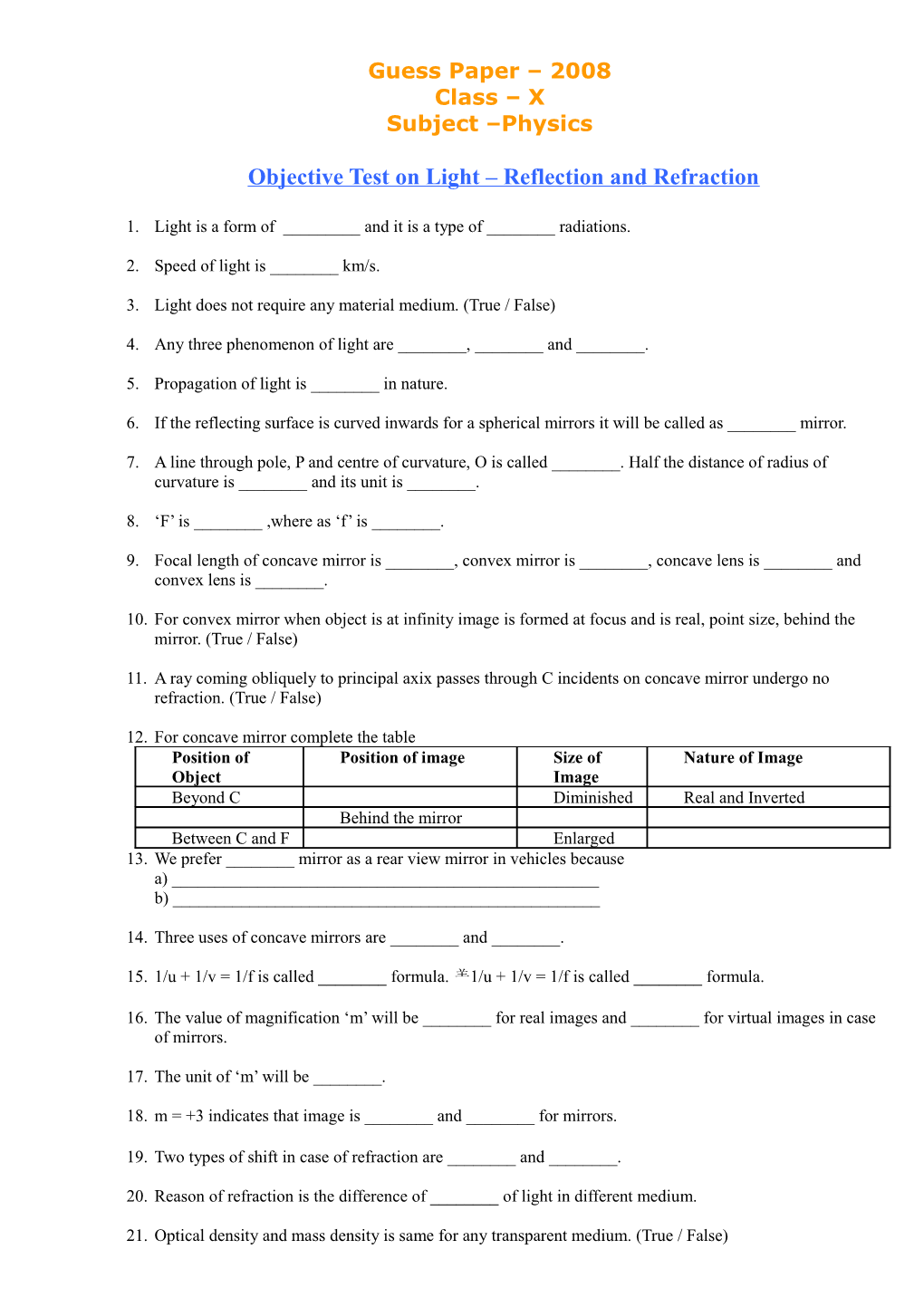# Objective Test on Light Reflection and RefractionGuess Paper – 2008
Class – X
Subject –Physics

Objective Test on Light – Reflection and Refraction

1. Light is a form of ______and it is a type of ______radiations.
2. Speed of light is ______km/s.
3. Light does not require any material medium. (True / False)
4. Any three phenomenon of light are ______, ______and ______.
5. Propagation of light is ______in nature.
6. If the reflecting surface is curved inwards for a spherical mirrors it will be called as ______mirror.
7. A line through pole, P and centre of curvature, O is called ______. Half the distance of radius of curvature is ______and its unit is ______.
8. ‘F’ is ______,where as ‘f’ is ______.
9. Focal length of concave mirror is ______, convex mirror is ______, concave lens is ______and convex lens is ______.
10. For convex mirror when object is at infinity image is formed at focus and is real, point size, behind the mirror. (True / False)
11. A ray coming obliquely to principal axix passes through C incidents on concave mirror undergo no refraction. (True / False)
12. For concave mirror complete the table

Position of Object / Position of image / Size of Image / Nature of Image
Beyond C / Diminished / Real and Inverted
Behind the mirror
Between C and F / Enlarged
1. We prefer ______mirror as a rear view mirror in vehicles because

a) ______

b) ______

1. Three uses of concave mirrors are ______and ______.
2. 1/u + 1/v = 1/f is called ______formula. 1/u + 1/v = 1/f is called ______formula.
3. The value of magnification ‘m’ will be ______for real images and ______for virtual images in case of mirrors.
4. The unit of ‘m’ will be ______.
5. m = +3 indicates that image is ______and ______for mirrors.
6. Two types of shift in case of refraction are ______and ______.
7. Reason of refraction is the difference of ______of light in different medium.
8. Optical density and mass density is same for any transparent medium. (True / False)
9. n12 indicates that light is traveling from medium ______to medium ______.
10. (sini) / (sinr) = ______and is called as ______of the medium where the light is reaching with respect to medium where from light is coming. Its unit is ______.
11. n21 = ------(write formula of speed).
12. Focal length for convex lens is ______and concave lens is ______.
13. Speed of light in water will be______
14. If f1 and f2 are the focal length of two lenses, then the focal length of combination will be______.
15. Power and focal length of thin sheet of glass will be ______and ______respectively.
16. A convex lens of power 4 is combined with concave lens of power 0.8 The power of combination will be ______.(with sign)
17. Doctor suggested me to wear a lens of power 1.5. My lens will have focal length = ______(in cms with sign)
18. An object of size 7 cm is placed at 27 cm in front of concave mirror of focal length 18 cm. Screen should be placed at a distance of ______from pole to get sharp image. The size and nature of image will beof image will be ______and ______respectively.
19. A convex lens produces an inverted image magnified three times of an object placed at a distance of 15 cm from it. Its focal length will be______(with sign)

♣ Marks: 60 ♣ Time Allotted: 70 minutes ♣